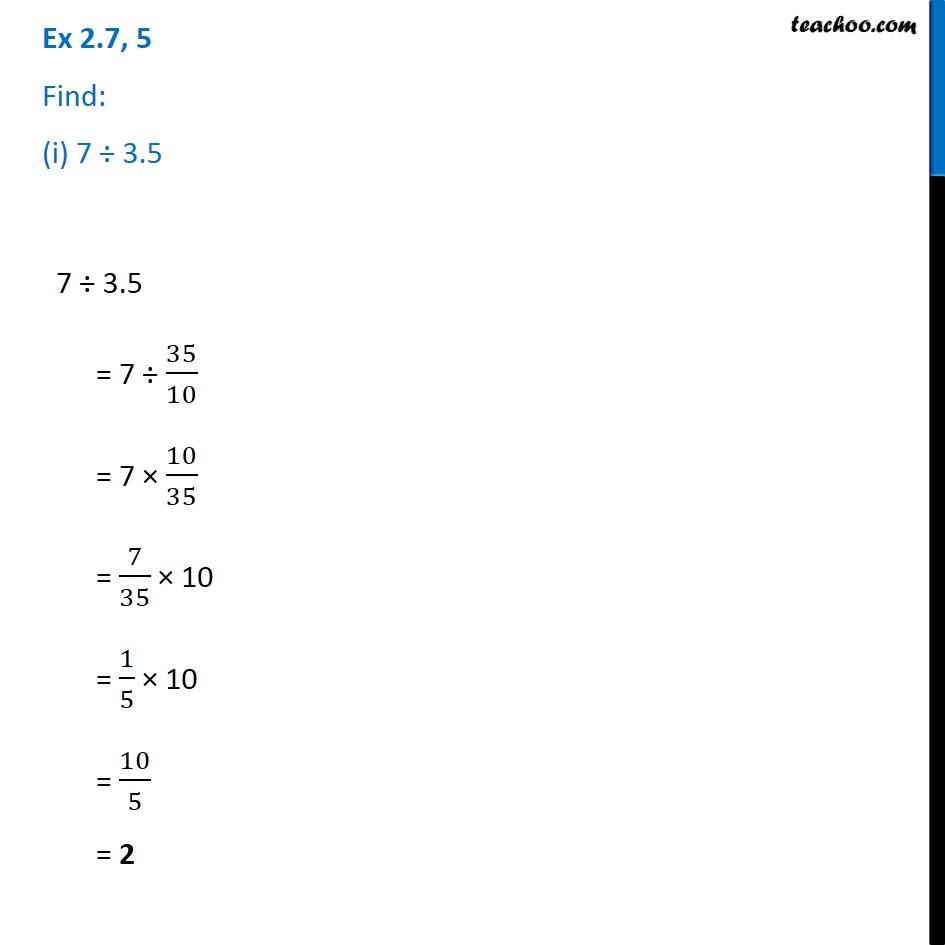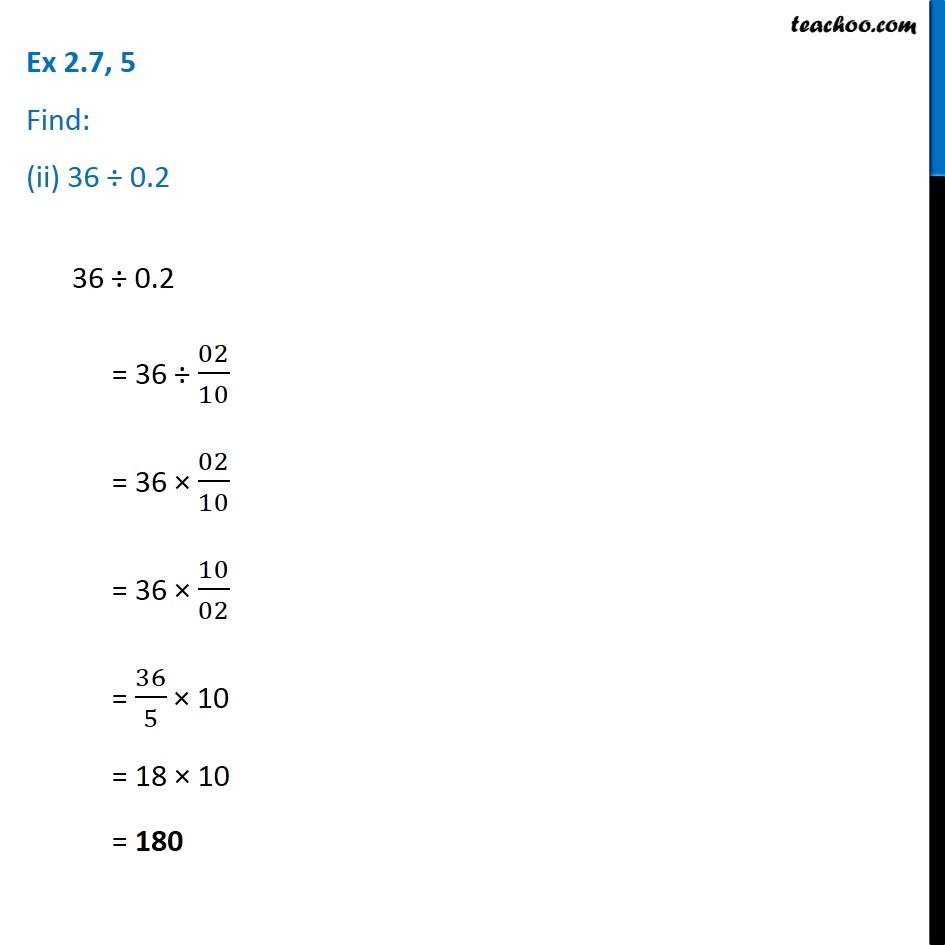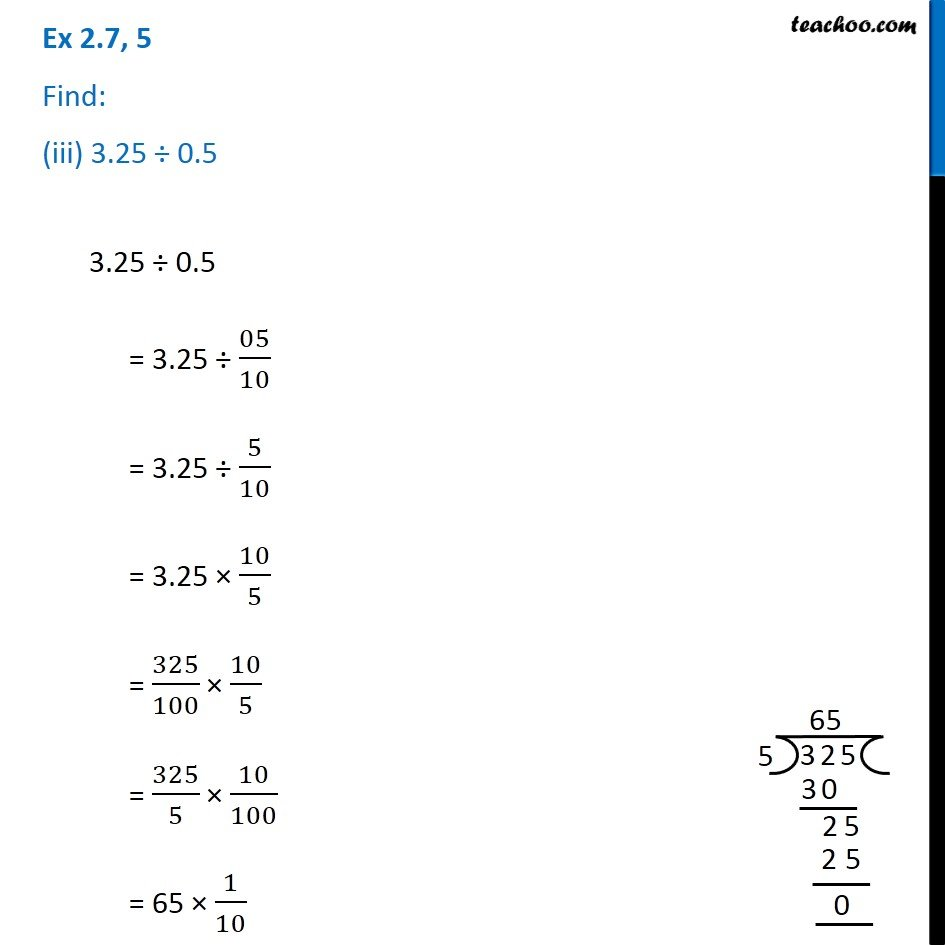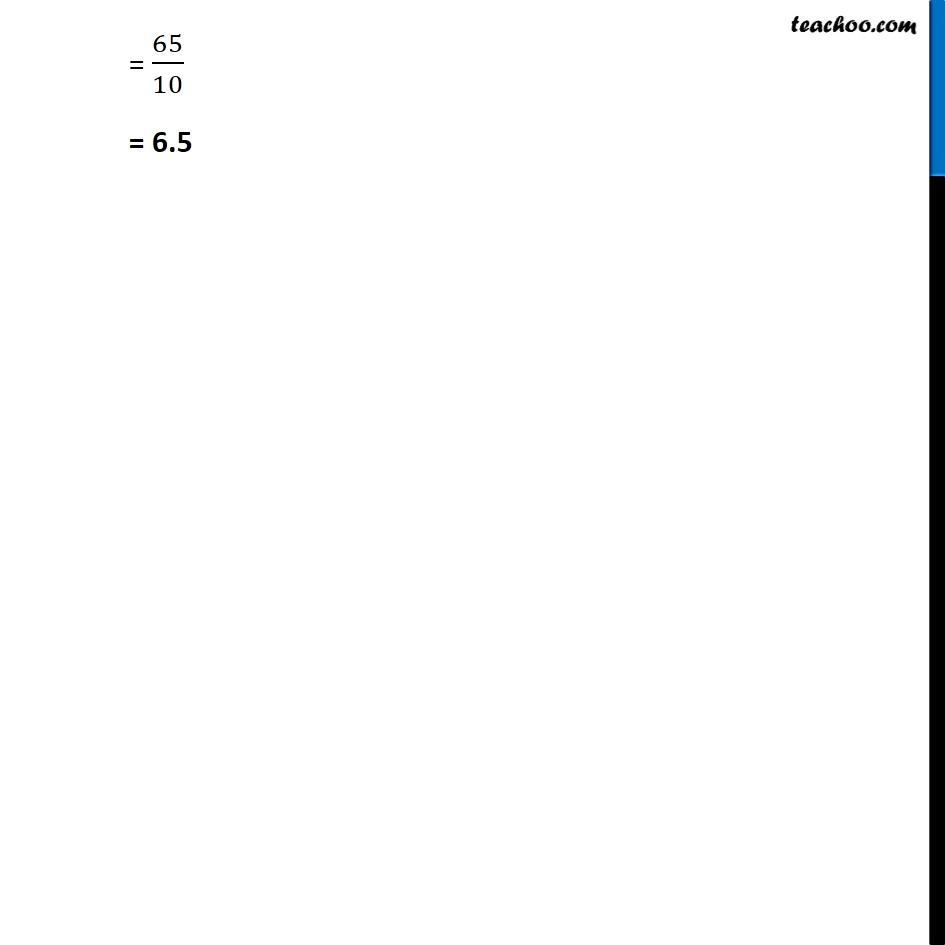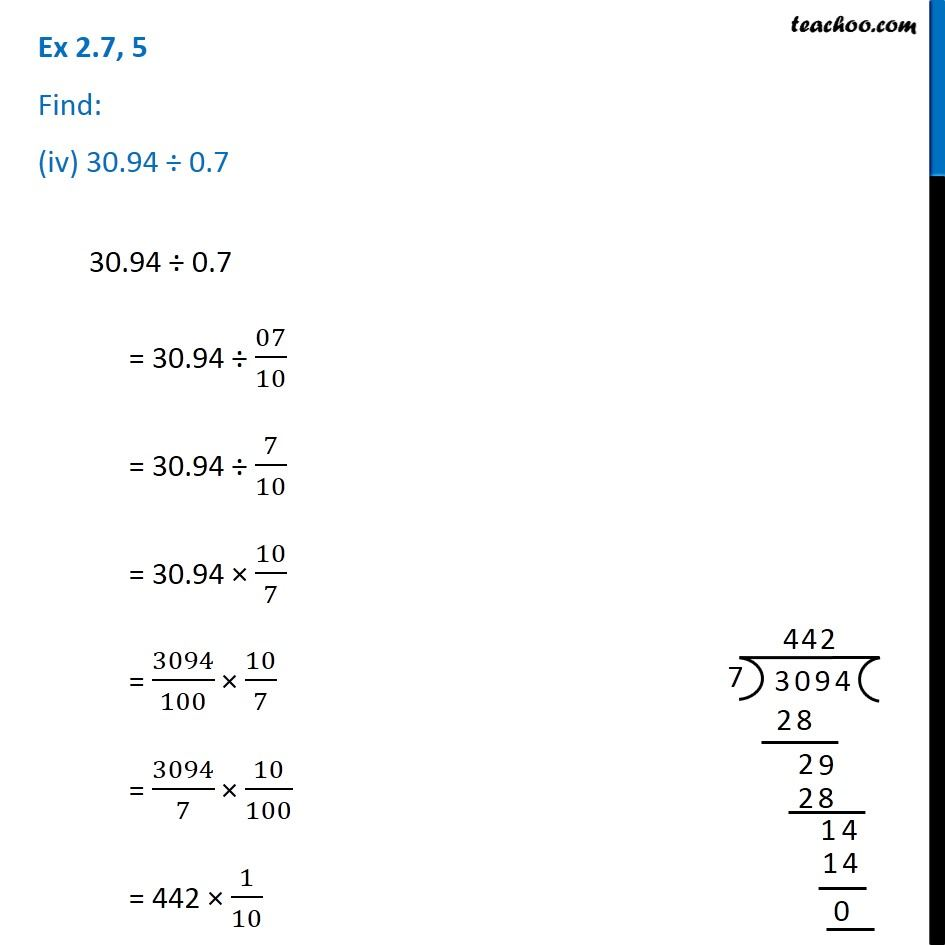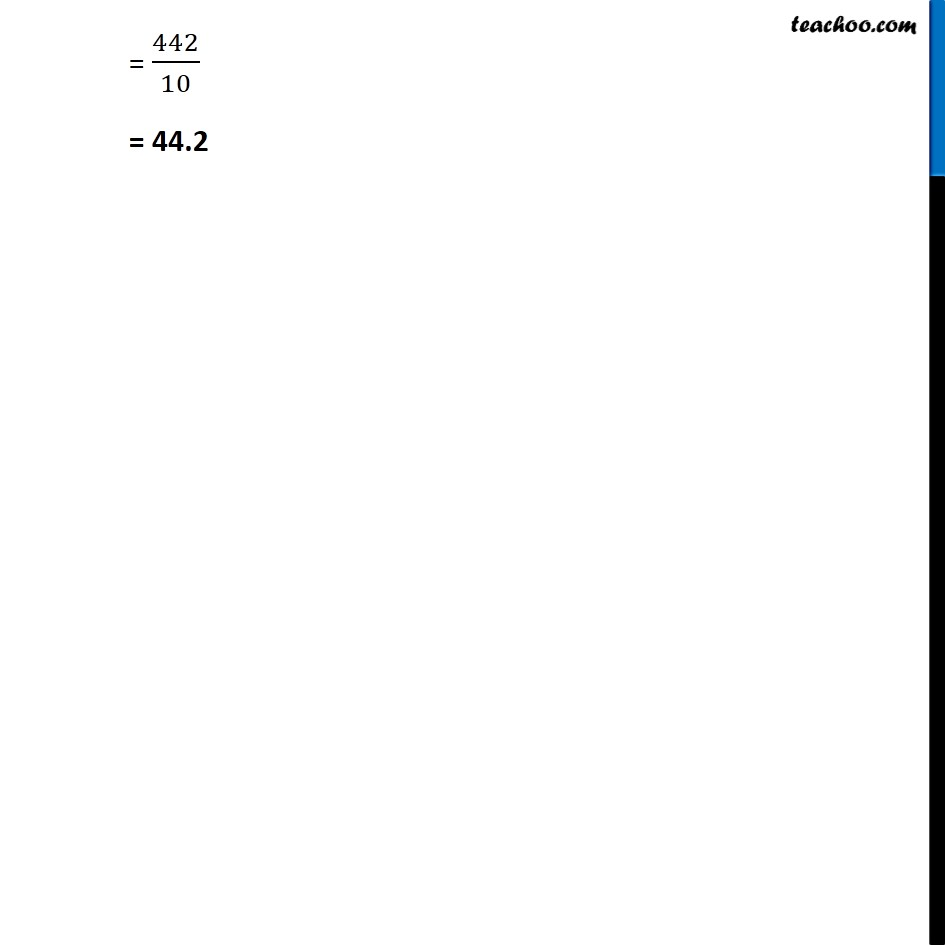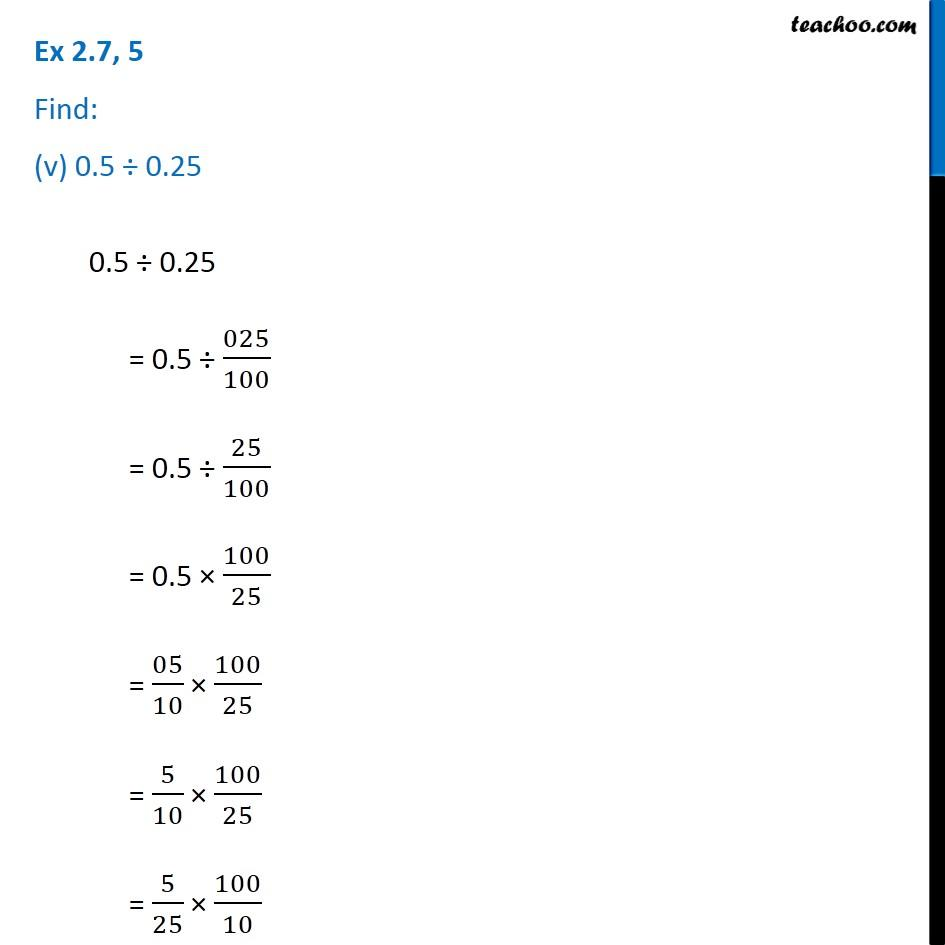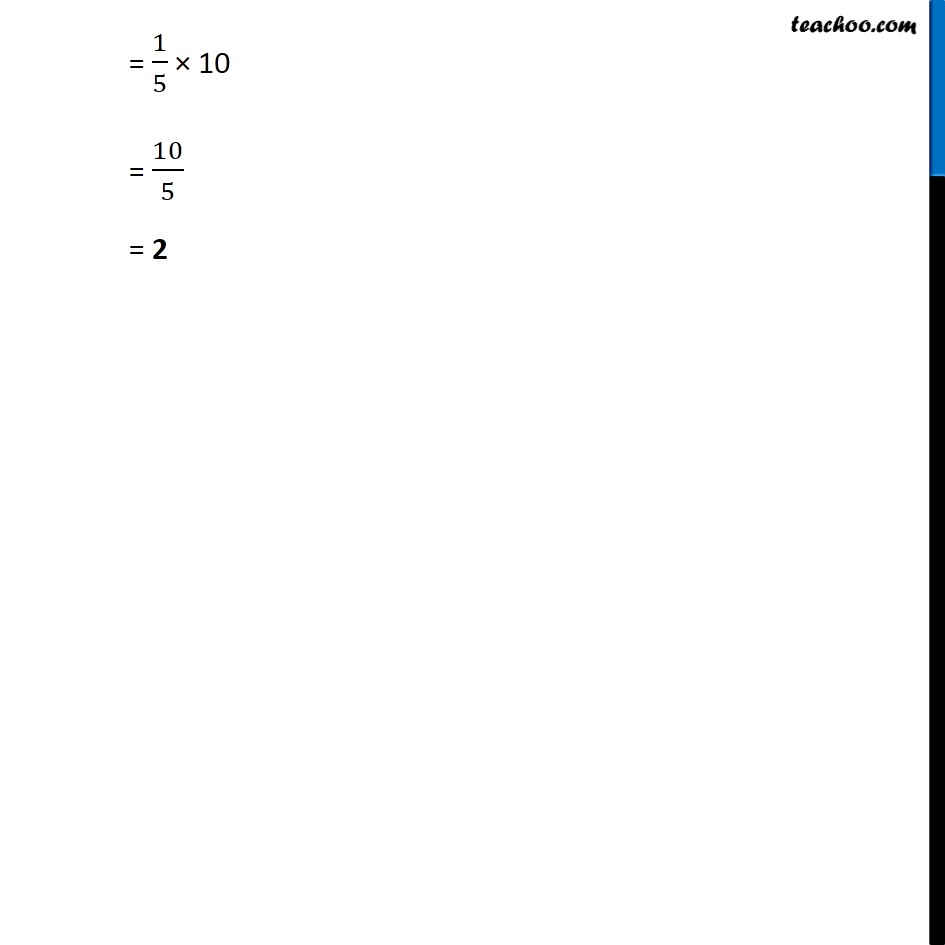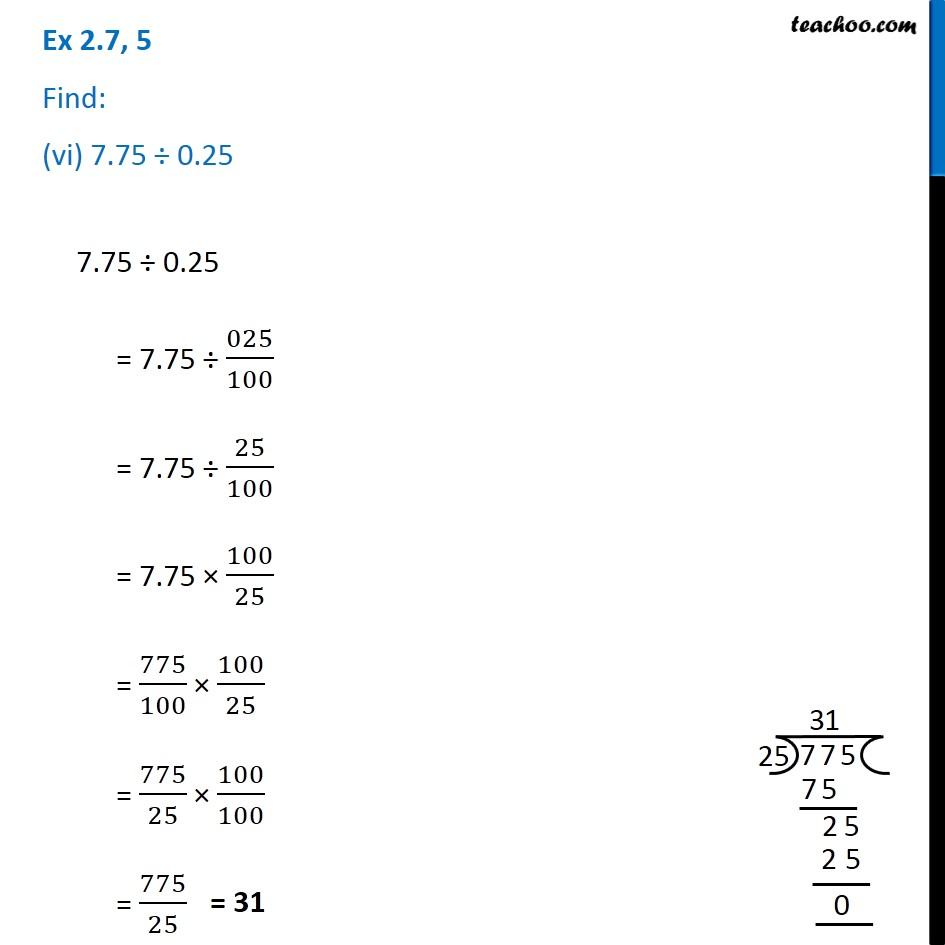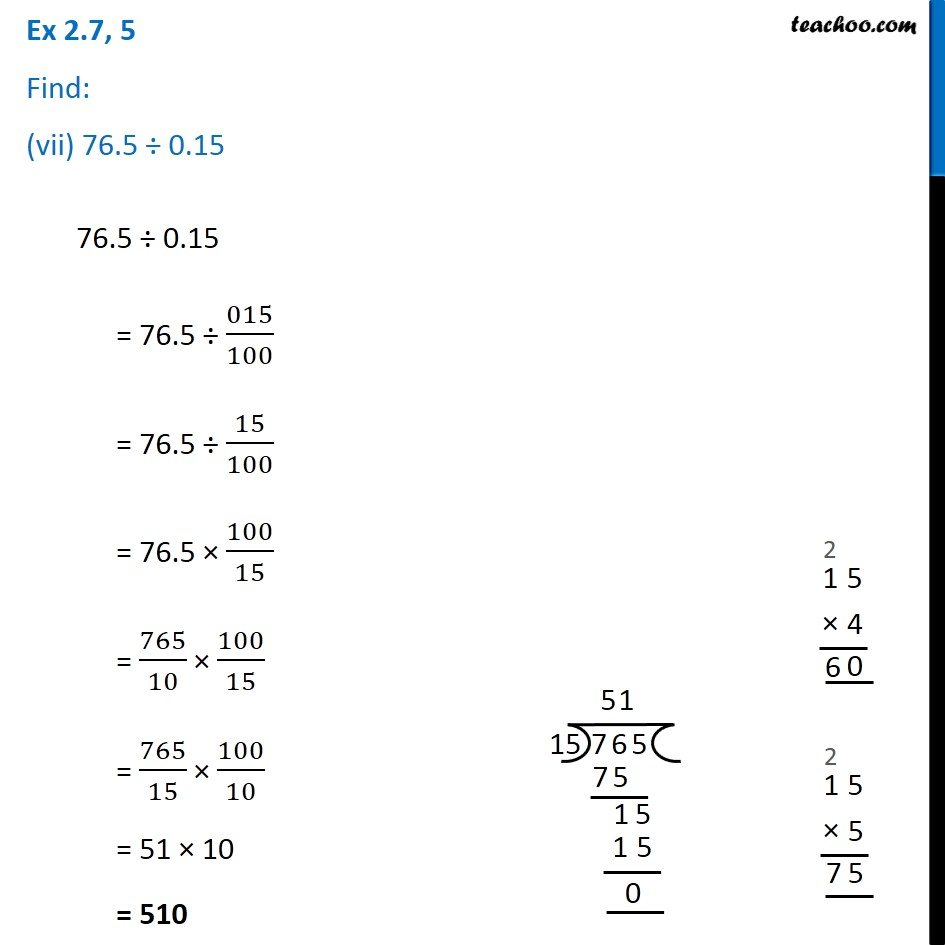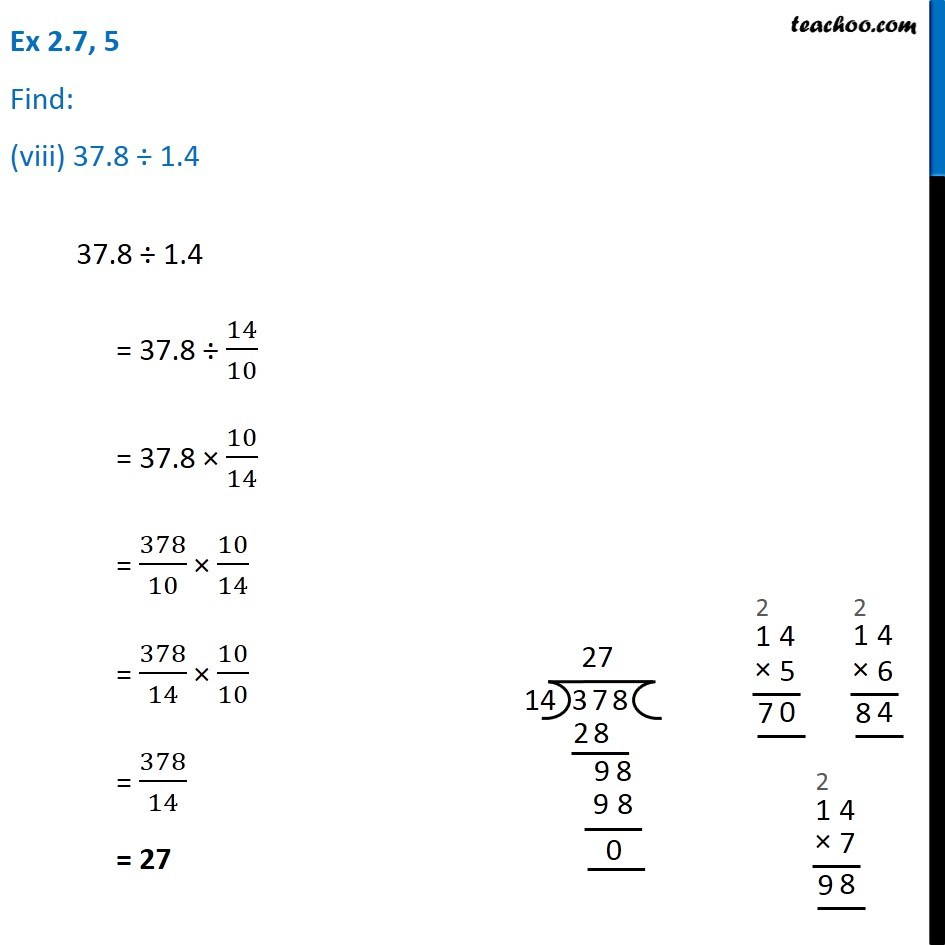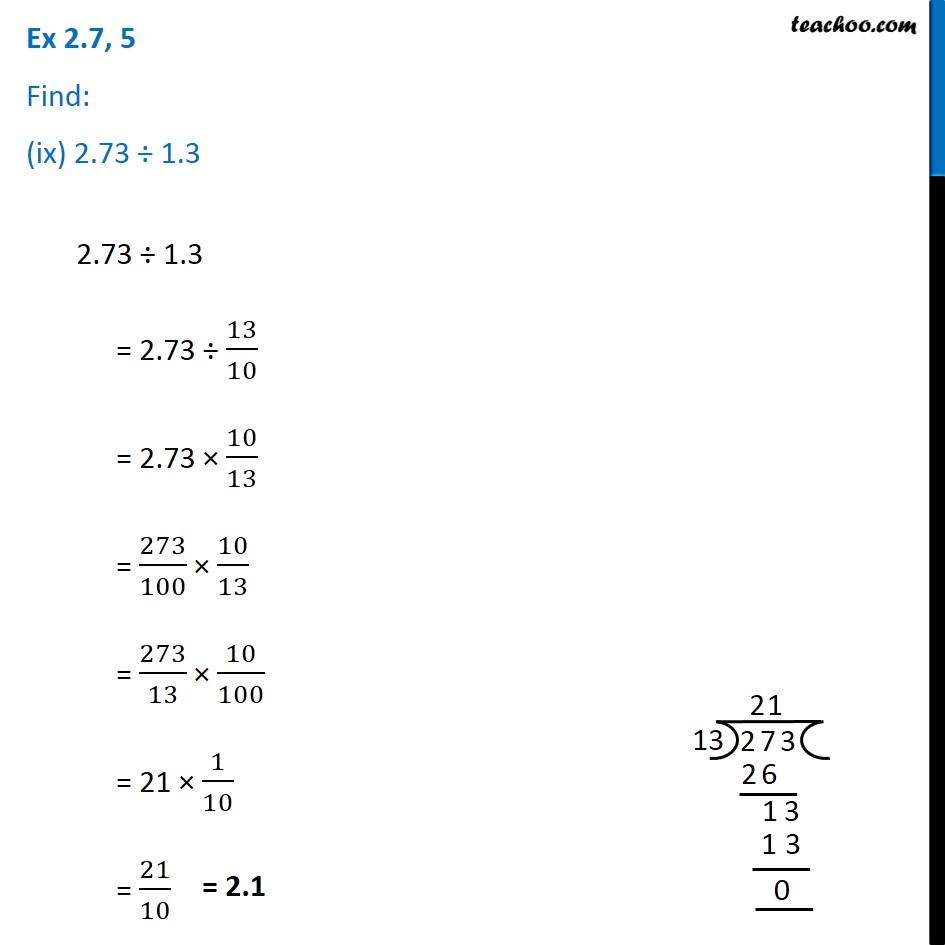1. Chapter 2 Class 7 Fractions and Decimals
2. Serial order wise
3. Ex 2.7

Transcript

Ex 2.7, 5 Find: (i) 7 ÷ 3.5 7 ÷ 3.5 = 7 35/10 = 7 10/35 = 7/35 10 = 1/5 10 = 10/5 = 2 Ex 2.7, 5 Find: (ii) 36 ÷0.2 36 0.2 = 36 02/10 = 36 02/10 = 36 10/02 = 36/5 10 = 18 10 = 180 Ex 2.7, 5 Find: (iii) 3.25 ÷ 0.5 3.25 ÷ 0.5 = 3.25 05/10 = 3.25 5/10 = 3.25 10/5 = 325/100 10/5 = 325/5 10/100 = 65 1/10 = 65/10 = 6.5 Ex 2.7, 5 Find: (iv) 30.94 ÷ 0.7 30.94 ÷ 0.7 = 30.94 07/10 = 30.94 7/10 = 30.94 10/7 = 3094/100 10/7 = 3094/7 10/100 = 442 1/10 = 442/10 = 44.2 Ex 2.7, 5 Find: (v) 0.5 0.250.5 0.25 = 0.5 025/100 = 0.5 25/100 = 0.5 100/25 = 05/10 100/25 = 5/10 100/25 = 5/25 100/10 = 1/5 10 = 10/5 = 2 Ex 2.7, 5 Find: (vi) 7.75 ÷ 0.25 7.75 ÷ 0.25 = 7.75 025/100 = 7.75 25/100 = 7.75 100/25 = 775/100 100/25 = 775/25 100/100 = 775/25 Ex 2.7, 5 Find: (vii) 76.5 ÷ 0.15 76.5 ÷ 0.15 = 76.5 015/100 = 76.5 15/100 = 76.5 100/15 = 765/10 100/15 = 765/15 100/10 = 51 10 = 510 Ex 2.7, 5 Find: (viii) 37.8 ÷ 1.4 37.8 ÷ 1.4 = 37.8 14/10 = 37.8 10/14 = 378/10 10/14 = 378/14 10/10 = 378/14 = 27 Ex 2.7, 5 Find: (ix) 2.73 ÷ 1.3 2.73 ÷ 1.3 = 2.73 13/10 = 2.73 10/13 = 273/100 10/13 = 273/13 10/100 = 21 1/10 = 21/10

Ex 2.7

About the AuthorDavneet Singh
Davneet Singh is a graduate from Indian Institute of Technology, Kanpur. He has been teaching from the past 10 years. He provides courses for Maths and Science at Teachoo.